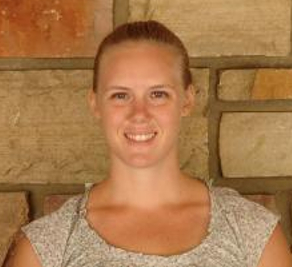# JEM N. CORCORAN

### ASSOCIATE PROFESSOR OF APPLIED MATHEMATICS

Professor Corcoran is one of the founding members of the Probability and Statistics Group in the Department of Applied Mathematics at CU Boulder. She works in the areas of sequential Monte Carlo methods, image processing, and the development and application Markov chain Monte Carlo algorithms.

## CONTACT ME

Phone : (303)492-0685

Address :526 UCB, Boulder, CO 80309

## Coupling from the Past for the Stochastic Simulation of Chemical Reaction Networks

### Submitted: May 12, 2021

Chemical reaction networks (CRNs) are fundamental computational models used to study the behavior of chemical reactions in well-mixed solutions. They have been used extensively to model a broad range of biological systems, and are primarily used when the more traditional model of deterministic continuous mass action kinetics is invalid due to small molecular counts. We present a perfect sampling algorithm to draw error-free samples from the stationary distributions of stochastic models for coupled, linear chemical reaction networks. ... The state spaces of such networks are given by all permissible combinations of molecular counts for each chemical species, and thereby grow exponentially with the numbers of species in the network. To avoid simulations involving large numbers of states, we propose a subset of chemical species such that coupling of paths started from these states guarantee coupling of paths started from all states in the state space and we show for the well-known Reversible Michaelis-Menten model that the subset does in fact guarantee perfect draws from the stationary distribution of interest. We compare solutions computed in two ways with this algorithm to those found analytically using the chemical master equation and we compare the distribution of coupling times for the two simulation approaches.

## Perfect Gibbs Sampling of Order Constrained Non-IID Ordered Random Variates with Application to Bayesian Principal Components Analysis

### Submitted: December 2020

Order statistics arising from m independent but not identically distributed random variables are typically constructed by arranging some X1,X2,…,Xm, with Xi having distribution function Fi(x), in increasing order denoted as X(1)≤X(2)≤…≤X(m). In this case, X(i) is not necessarily associated with Fi(x). Assuming one can simulate values from each distribution, one can generate such non-iid" order statistics by simulating Xi from Fi, for i=1,2,…,m, and simply putting them in order. In this paper, we consider the problem of simulating ordered values X(1),X(2),…,X(m) such that the marginal distribution of X(i) is Fi(x). ...This problem arises in Bayesian principal components analysis (BPCA) where the Xi are ordered eigenvalues that are a posteriori independent but not identically distributed. In this paper, we propose a novel {\emph{coupling-from-the-past}} algorithm to perfectly" (up to computable order of accuracy) simulate such "order-constrained non-iid" order statistics. We demonstrate the effectiveness of our approach for several examples, including the BPCA problem.

## Bayesian Fusion of Data Partitioned Particle Estimates

### Submitted: October 2020

We present a Bayesian data fusion method to approximate a posterior distribution from an ensemble of particle estimates that only have access to subsets of the data. Our approach relies on approximate probabilistic inference of model parameters through Monte Carlo methods, followed by an update and resample scheme related to multiple importance sampling to combine information from the initial estimates. We show the method is convergent in the particle limit and directly suited to application on multi-sensor data fusion problems by demonstrating efficacy on a multi-sensor Keplerian orbit determination problem and a bearings-only tracking problem.

## Current Students## Caleb Miller

MCMC, Bayesian Inference, Particle Filtering, Target Tracking, Data Fusion## Joy Mueller

MCMC, Chemical Kinetic Networks, Image Processing, Level Set Methods## Lilac Intrater

Markov Processes, Queueing, Jackson Networks

Coming soon.

## Fall 2021

Mathematical Statistics STAT 5530

## Previous

Mathematical Statistics • Markov Processes • Measure Theoretic Probability • Stochastic Simulation • Stochastic Differential Equations • Bayesian Statistics • Applied Analysis • Time Series